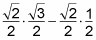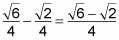##### Pre-Calculus All-in-One For DummiesYou can use the concept of sum and difference formulas to calculate the sine of special angles in radians. This process is different than solving equations because here you're asked to find the trig value of a specific angle that isn't readily marked on the unit circle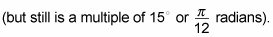Prior to choosing the appropriate formula, you simply break the angle into either the sum or the difference of two angles from the unit circle. Refer to the unit circle and notice the angles in radians in the figure.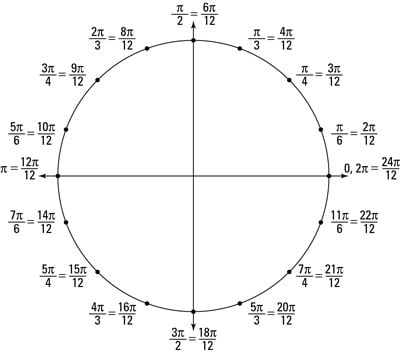The unit circle showing angles in radians with common denominators

You see that all the denominators are different, which makes adding and subtracting them a nightmare. You must find a common denominator so that adding and subtracting is a dream. The common denominator is 12, as you can see in the figure.

This figure comes in handy only for sum and difference formulas, because finding a common denominator is something you do only when you're adding or subtracting fractions.

As an example, follow these steps to find the exact value of sin(pi/12):

1. Rewrite the angle in question, using the special angles in radians with common denominators.

From the figure, you want a way to add or subtract two angles so that, in the end, you get pi/12.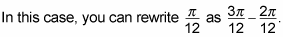2. Choose the appropriate sum or difference formula: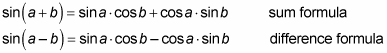Because you rewrote the angle with subtraction, you need to use the difference formula.

3. Plug the information you know into the chosen formula.

You know the following equality: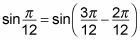Substitute as follows into the difference formula: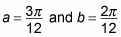Which gives you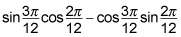4. Reduce the fractions in the formula to ones you're more comfortable with.

In this example, you can reduce to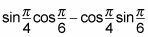Now you'll have an easier time referring to the unit circle to get your equation.

5. Use the unit circle to look up the sine and cosine values that you need.

You now have